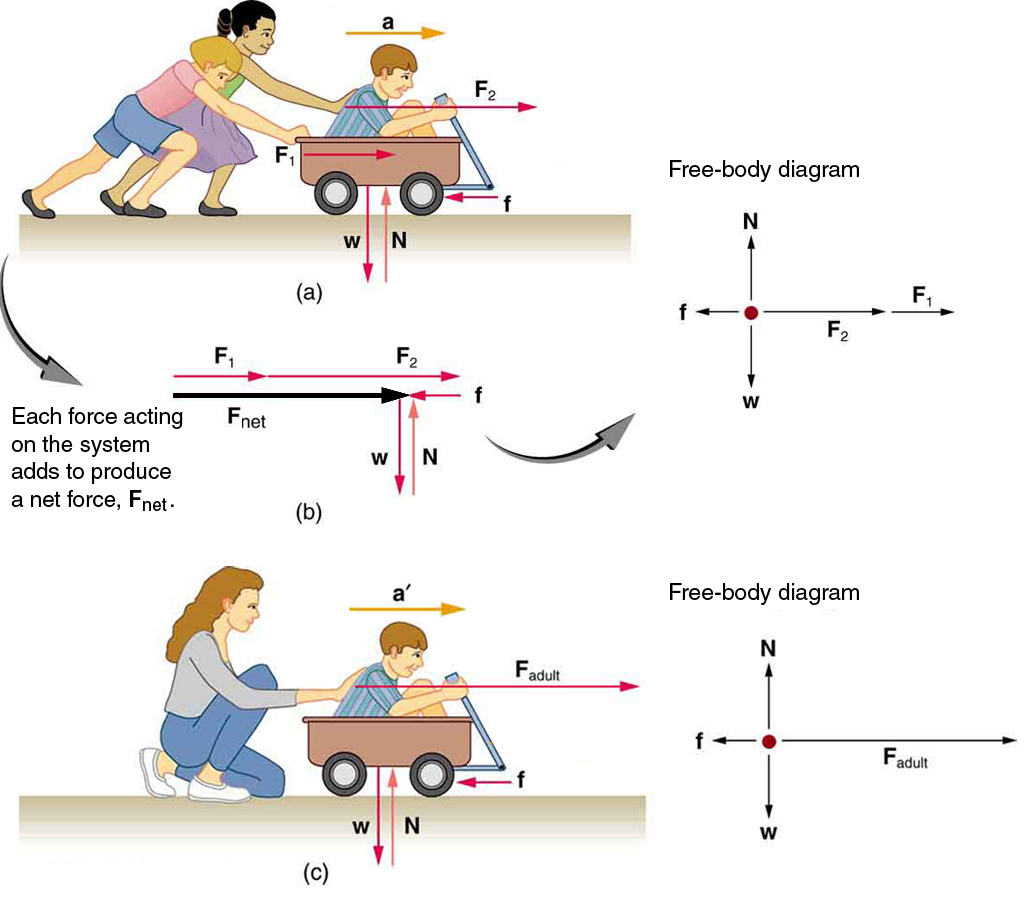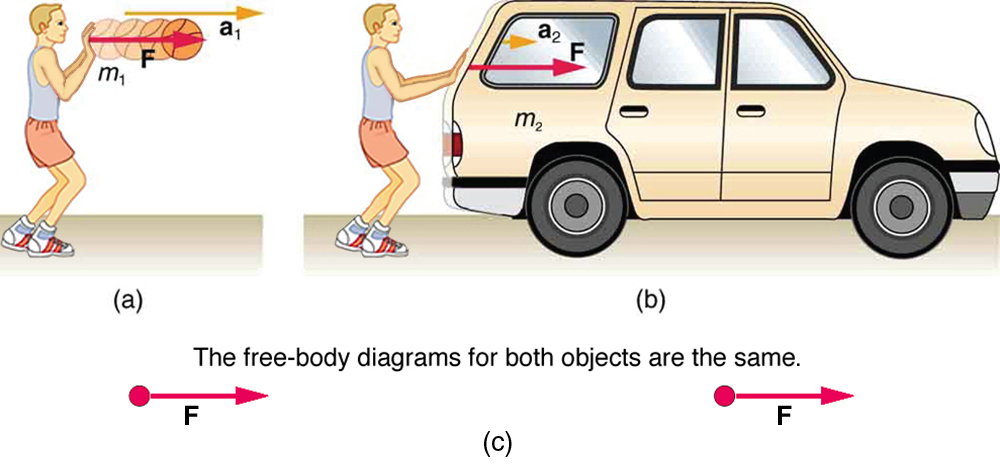# Newtons Second law of motions

Introduction

Good morning learners, Today we start a new lesson which is Newtons second law of motion.

## What is Newton's second Law?

In the world of introductory physics, Newton's second law is one of the most important laws you'll learn. It's used in almost every chapter of every physics textbook, so it's important to master this law as soon as possible.

We know objects can only accelerate if there are forces on the object. Newton's second law tells us exactly how much an object will accelerate for a given net force.

.Watch the follow video so that you may understand the key concepts of this lesson.

Watch the following video and try to answer the following questions

September 2021

total marks 20 marks

Class Activity

Question 1

Two crates, 10 kg and 15 kg respectively, are connected with a thick rope according
to the diagram. A force, to the right, of 500 N is applied. The boxes move with anacceleration of 2 m·s−2 to the right. One third of the total frictional force is acting on the 10 kg block and two thirds on the 15 kg block. Calculate:

1. the magnitude and direction of the total frictional force present.
2. the magnitude of the tension in the rope at T.

Questions 2

A 10 kg box is placed on a table. A horizontal force of magnitude 32 N is applied to
the box. A frictional force of magnitude 7 N is present between the surface and the
box.

1. Draw a force diagram indicating all of the forces acting on the box.
2. Calculate the acceleration of the box.
Process

Please watch this video learners so that you may understand the key concepts of Newtons second law of motions. After watching the video there are notes provided.

Newton’s second law of motion is closely related to Newton’s first law of motion. It mathematically states the cause and effect relationship between force and changes in motion. Newton’s second law of motion is more quantitative and is used extensively to calculate what happens in situations involving a force. Before we can write down Newton’s second law as a simple equation giving the exact relationship of force, mass, and acceleration, we need to sharpen some ideas that have already been mentioned.

First, what do we mean by a change in motion? The answer is that a change in motion is equivalent to a change in velocity. A change in velocity means, by definition, that there is an acceleration. Newton’s first law says that a net external force causes a change in motion; thus, we see that a net external force causes acceleration.

Another question immediately arises. What do we mean by an external force? An intuitive notion of external is correct—an external force acts from outside the system of interest. For example, in (Figure)(a) the system of interest is the wagon plus the child in it. The two forces exerted by the other children are external forces. An internal force acts between elements of the system. Again looking at (Figure)(a), the force the child in the wagon exerts to hang onto the wagon is an internal force between elements of the system of interest. Only external forces affect the motion of a system, according to Newton’s first law. (The internal forces actually cancel, as we shall see in the next section.) You must define the boundaries of the system before you can determine which forces are external. Sometimes the system is obvious, whereas other times identifying the boundaries of a system is more subtle. The concept of a system is fundamental to many areas of physics, as is the correct application of Newton’s laws. This concept will be revisited many times on our journey through physics.

Different forces exerted on the same mass produce different accelerations. (a) Two children push a wagon with a child in it. Arrows representing all external forces are shown. The system of interest is the wagon and its rider. The weightof the system and the support of the groundare also shown for completeness and are assumed to cancel. The vectorrepresents the friction acting on the wagon, and it acts to the left, opposing the motion of the wagon. (b) All of the external forces acting on the system add together to produce a net force,. The free-body diagram shows all of the forces acting on the system of interest. The dot represents the center of mass of the system. Each force vector extends from this dot. Because there are two forces acting to the right, we draw the vectors collinearly. (c) A larger net external force produces a larger acceleration () when an adult pushes the child.Now, it seems reasonable that acceleration should be directly proportional to and in the same direction as the net (total) external force acting on a system. This assumption has been verified experimentally and is illustrated in (Figure). In part (a), a smaller force causes a smaller acceleration than the larger force illustrated in part (c). For completeness, the vertical forces are also shown; they are assumed to cancel since there is no acceleration in the vertical direction. The vertical forces are the weightand the support of the ground, and the horizontal forcerepresents the force of friction. These will be discussed in more detail in later sections. For now, we will define friction as a force that opposes the motion past each other of objects that are touching. (Figure)(b) shows how vectors representing the external forces add together to produce a net force,.

To obtain an equation for Newton’s second law, we first write the relationship of acceleration and net external force as the proportionalitywhere the symbolmeans “proportional to,” andis the net external force. (The net external force is the vector sum of all external forces and can be determined graphically, using the head-to-tail method, or analytically, using components. The techniques are the same as for the addition of other vectors, and are covered in Two-Dimensional Kinematics.) This proportionality states what we have said in words—acceleration is directly proportional to the net external force. Once the system of interest is chosen, it is important to identify the external forces and ignore the internal ones. It is a tremendous simplification not to have to consider the numerous internal forces acting between objects within the system, such as muscular forces within the child’s body, let alone the myriad of forces between atoms in the objects, but by doing so, we can easily solve some very complex problems with only minimal error due to our simplification

Now, it also seems reasonable that acceleration should be inversely proportional to the mass of the system. In other words, the larger the mass (the inertia), the smaller the acceleration produced by a given force. And indeed, as illustrated in (Figure), the same net external force applied to a car produces a much smaller acceleration than when applied to a basketball. The proportionality is written aswhereis the mass of the system. Experiments have shown that acceleration is exactly inversely proportional to mass, just as it is exactly linearly proportional to the net external force.

The same force exerted on systems of different masses produces different accelerations. (a) A basketball player pushes on a basketball to make a pass. (The effect of gravity on the ball is ignored.) (b) The same player exerts an identical force on a stalled SUV and produces a far smaller acceleration (even if friction is negligible). (c) The free-body diagrams are identical, permitting direct comparison of the two situations. A series of patterns for the free-body diagram will emerge as you do more problems.It has been found that the acceleration of an object depends only on the net external force and the mass of the object. Combining the two proportionalities just given yields Newton’s second law of motion.

Newton’s Second Law of Motion

The acceleration of a system is directly proportional to and in the same direction as the net external force acting on the system, and inversely proportional to its mass.

In equation form, Newton’s second law of motion isThis is often written in the more familiar formWhen only the magnitude of force and acceleration are considered, this equation is simplyResources

More examples of Newtons second law of motions : https://byjus.com/questions/list-some-examples-of-newtons-second-law-of-motion/

Newtons second law of motions pratical examples: https://www.indypl.org/blog/for-kids/science-experiment-newtons-second-law-of-motion

The following video tells more about acceleration.

Evaluation
 Question 1 3 marks 7 marks Question 2 5 marks 5 marks total 20 marks

Conclusion

These videos and the task will help you prepare for you coming term test so make sure you watch the videos carefully. you have a 4 days to complete the class Activity and your activity is due on the 4 September 2021.

Good luck.

Teacher Page

Anathi Siwendu

Student number : 218315589